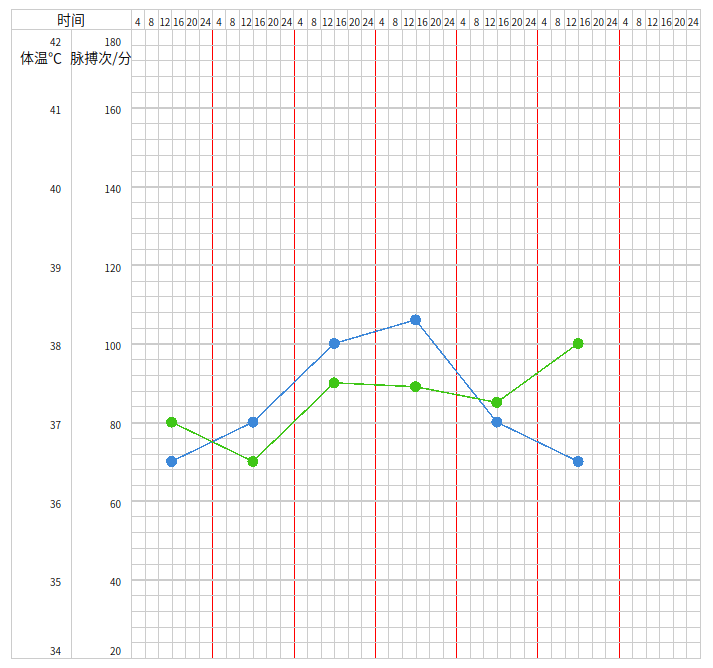# d3.js实现自定义多y轴折线图的示例代码1.初始化一个svg作为容器，之后所有的点线面都是在这个容器里边画了

``````
svg.select('#id')
.append('svg')
.attr('width', width)
.attr('height', height)
``````

2.定义比例尺 `scale `，定义域 `domain `显示的刻度范围,值域 `range `实际数据刻度

``````
// x轴以时间为刻度
this.x = d3
.scaleTime()
.domain([this.beginTime, this.endTime])
.range([0, this.width]);

// y轴按照像素值为刻度，所有数据需按照比例转换计算
this.y = d3
.scaleLinear()
.domain([0, this.height])
.range([this.height, 0]);

``````

3.定义轴 `axis `, `axis `需要和 `scale `结合使用，作为参数传入 `axis(scale)`

``````
this.xAxis = d3
.axisTop(this.x)
// .ticks(d3.timeHour.every(4))
.tickValues(d3.timeHour.range(this.beginTime, this.endTime, 4))
.tickSize(this.height)
.tickFormat(function (d, i) {
// return d.getHours();
return;
});

this.yAxis = d3
.axisLeft()
.scale(this.y)
.tickValues(d3.range(0, this.height, this.height / 40))
.tickSize(-this.width)
.tickFormat(function (d, i) {
return;
});

``````

4.定义折线模板

``````
// 折线模板
this.line = d3
.line()
.x(function (d) {
return that.x(d.datetime);
})
.y(function (d) {
return that.y(d.svgValue);
});
``````

5.以上就完成了画布的基本框架，剩下的事情就是数据渲染。

d3.js 多y轴折线图 d3.js 折线图